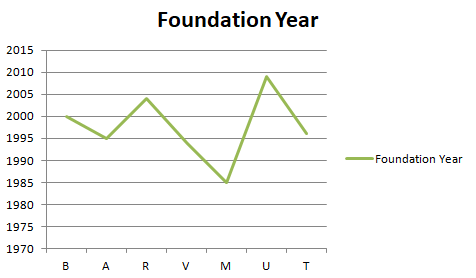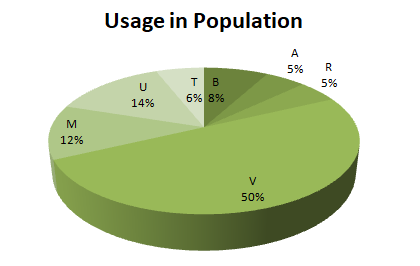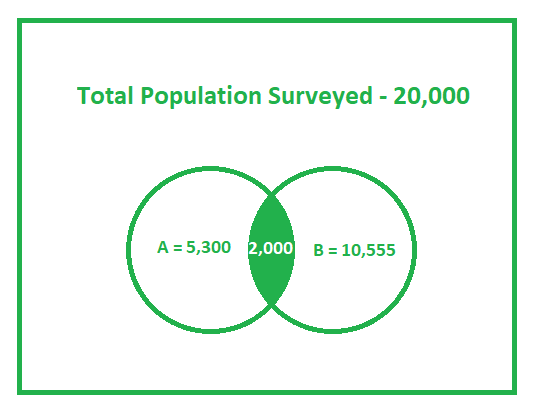# GRE Data Analysis | Data Interpretation Examples

• Last Updated : 09 Jul, 2019

Data Interpretation simply means to understand given data and transforming the same data into the desired property. Data given can be in any form from below given data representations

• Table
• Circular Graph
• Venn Diagram
• Bar Graph
• Scattered Plots
• Line Graphs

Approximately three Data Interpretation questions per section are asked in GRE and it can be of any type either MCQ or Numeric Entry.

### Data represented in Table Form:

The table shows a comparison of two plans(call charges for 1 minute and call charges for 2 minutes) provided by telecom companies. Please answer questions as per the table.

Telecom CompaniesCall Charge per minuteCall Charge per 2 minutes
B0.941
A0.981
R0.980.98
V0.950.98
M22.2
U1.11.3
T1.82.0

Ques-1:
Which company provides highest percentage increase in call charges from one minute plan to two minutes plan ?

Explanation:
For B, the Percentage increase = 0.06/0.94 = 6/946% (slightly greater than 6%)
For A, the Percentage increase = 0.02/0.98 = 2/982% (Slightly greater than 2%)
For R, there is no increase in the rates.
For V, the Percentage Increase = 0.03/0.95 = 3/953% (Slightly greater than 3%)
For M, the Percentage Increase = 0.2/2 = 1/10 = 10%
For U, the Percentage Increase = 0.2/1.1 = 2/1120% (Slightly less than 20%)
For T, the Percentage Increase = 0.2/1.8 = 2/18 = 11%

So the answer is U with 20% increase.

Ques-2:
How many companies increased charges in second plan greater than 10% ?

Explanation:
For B, the Percentage increase = 0.06/0.94 = 6/946% (slightly greater than 6%)
For A, the Percentage increase = 0.02/0.98 = 2/982% (Slightly greater than 2%)
For R, there is no increase in the rates.
For V, the Percentage Increase = 0.03/0.95 = 3/953% (Slightly greater than 3%)
For M, the Percentage Increase = 0.2/2 = 1/10 = 10%
For U, the Percentage Increase = 0.2/1.1 = 2/1120% (Slightly less than 20%)
For T, the Percentage Increase = 0.2/1.8 = 2/18 = 11%

Ques-3:
Based on the information in the table, which of the following three statements are true?

Explanation:
Statement 1: Percentage increase in charges of the second plan of M and T are the same.
Statement 2: M provides costly services amongst them all.
Statement 3: All companies provide less than 20% difference in both the plans.

• Statement-1: FALSE
Bases are different in both cases. Quantity with the higher base provides more percentage increase. In our case, it is M. Thus the statement is False.
• Statement-2: TRUE
Percentage increase, as well as the base of M, are highest. Thus it provides costly service amongst them all.
• Statement-3: TRUE
Increase in M rates are highest and also the percentage increase is highest which is slightly less than 20. Thus the statement is True.

### Data represented in Circular Graph and Line Graph:Above is mentioned the graph of Foundation years of different Telecom companies in a particular area and their usage in particular section. The total population of the section is 5, 00, 000.

Ques-1:
How many people use oldest telecom services?

Explanation:
The oldest telecom company is M having been founded in 1985.
From the pie chart, we can say that 12% of the population use telecom services provided by M.
Thus the answer is = 5, 00, 000 * (12/100) = 60, 000

Ques-2:
The companies founded after 2000 cover how much percentage of population ?

Explanation:
There are only two companies founded after 2000, which are R and U. So, the percentage of the population covered by these companies are (14 + 5) = 19%

### Data represented in Venn Diagram:

In a survey of 20, 000 people, 5, 300 have used telecom services provided by A; 10, 555 used telecom services provided by B and 2, 000 people have used services provided by both the providers.Ques-1:
How many people are using telecom services provided by A but not B ?

Explanation:
People using services provided by A – People using services provided by both

```= 5300 – 2000
= 3300 ```

Ques-2:
How many people are using services provided by at least one service provider ?

Explanation:
People using services provided by A + People using services provided by B – People using services provided by both

```= 5300 + 10555 – 2000
= 13855 ```

Ques-3:
How many people do not use services provided by either of the service providers ?

Explanation:
Total – People using at least one service,

```= 20000 – 13855
= 6145 ```
My Personal Notes arrow_drop_up